# RD Sharma Solutions For Class 7 Maths Chapter 14 Lines And Angles Exercise 14.2

The materials of RD Sharma Solutions for Class 7 Maths Exercise 14.2 of Chapter 14, in the form of PDF, is available here. Students who want to score high in Maths can download the PDF from the given links. Our expert team has formulated RD Sharma Solutions for Class 7 to help and fulfill the dreams of students. This exercise has thirty questions based on parallel lines and transversal lines. Let us have a look at some of the important concepts that are being discussed in this exercise.

• Definition and meaning of parallel lines
• Parallel rays
• Parallel segments
• Transversals
• Angle made by a transversal with two lines
• Exterior and interior angles
• Corresponding angles
• Alternate interior and interior angles
• Angles made by a transversal to two parallel lines

## Download the PDF of RD Sharma Solutions For Class 7 Maths Chapter 14 – Lines And Angles Exercise 14.2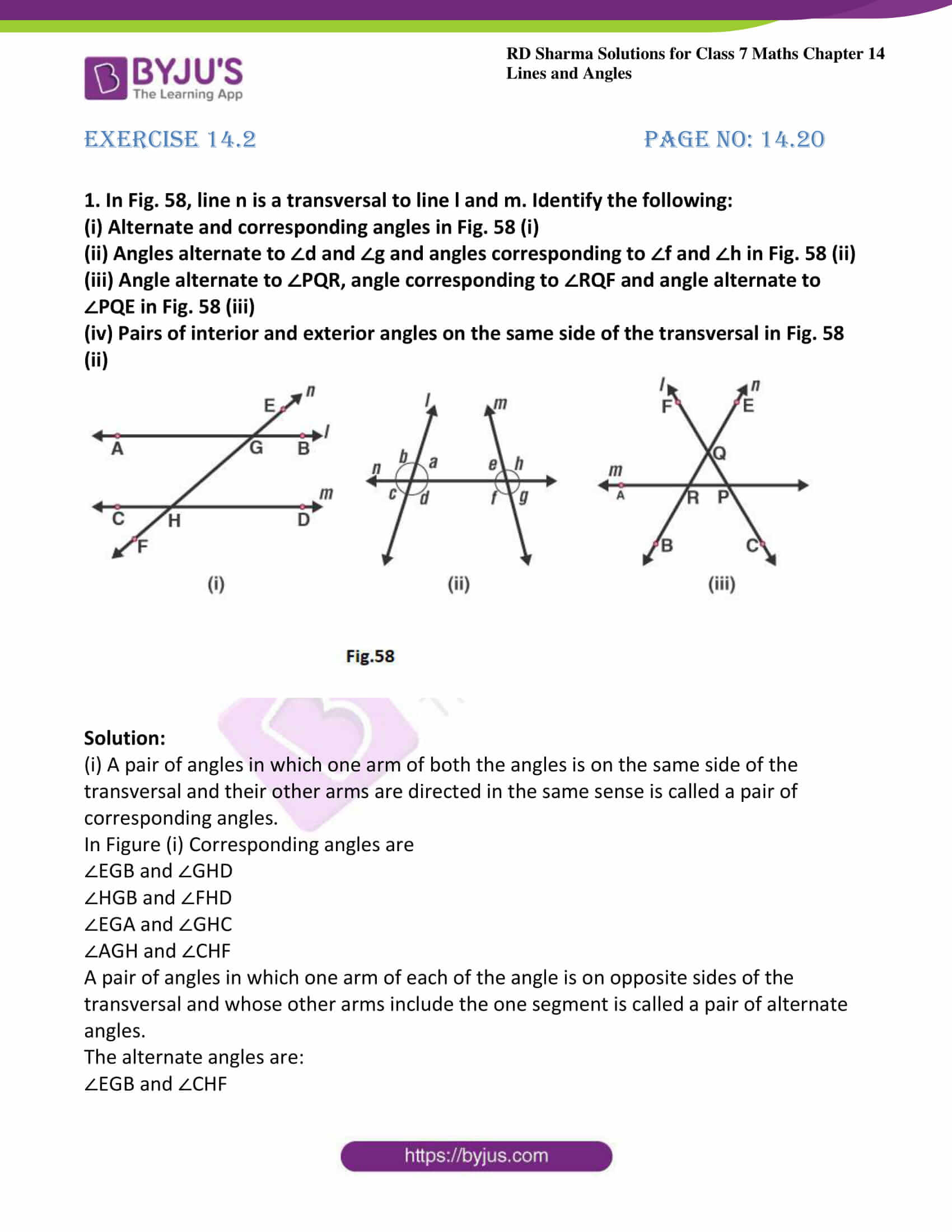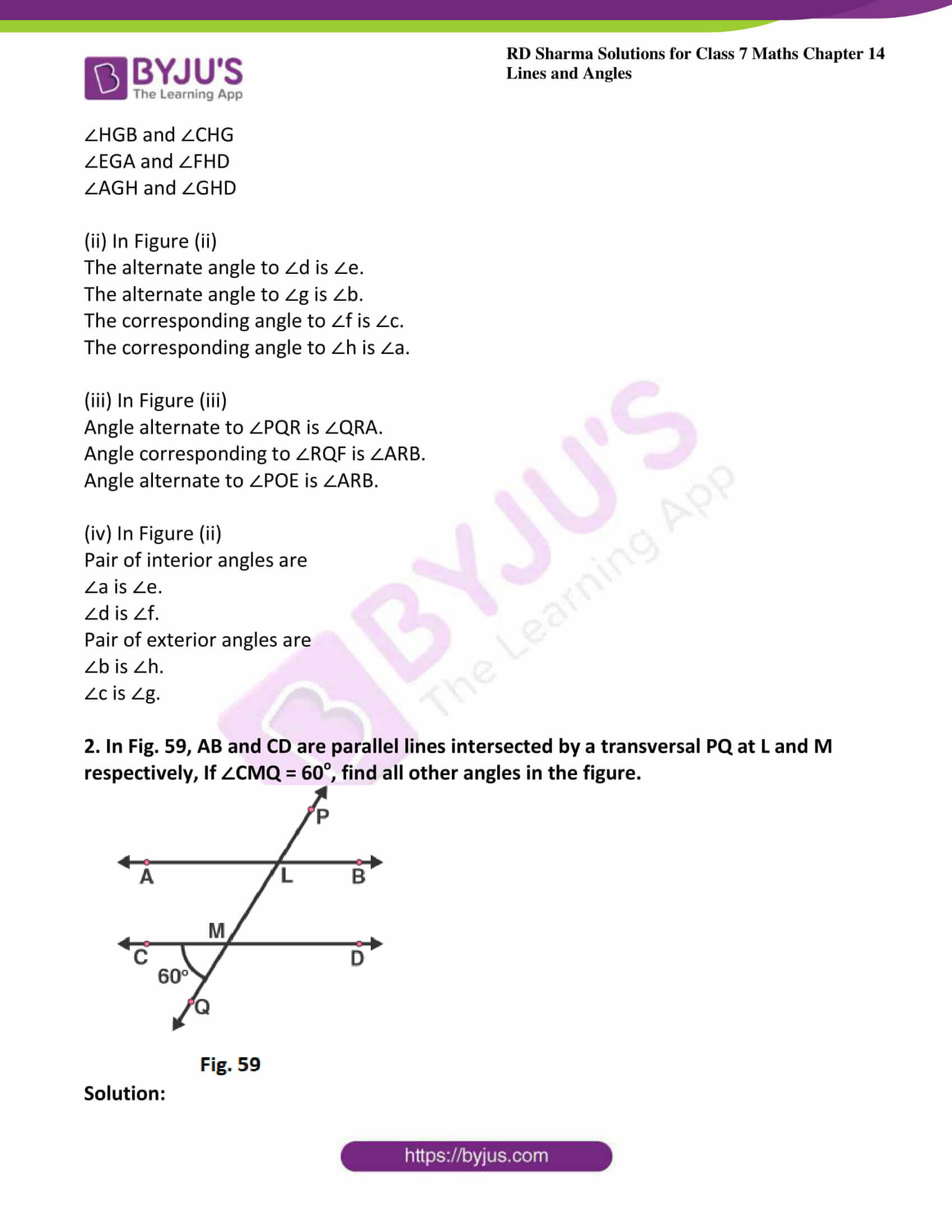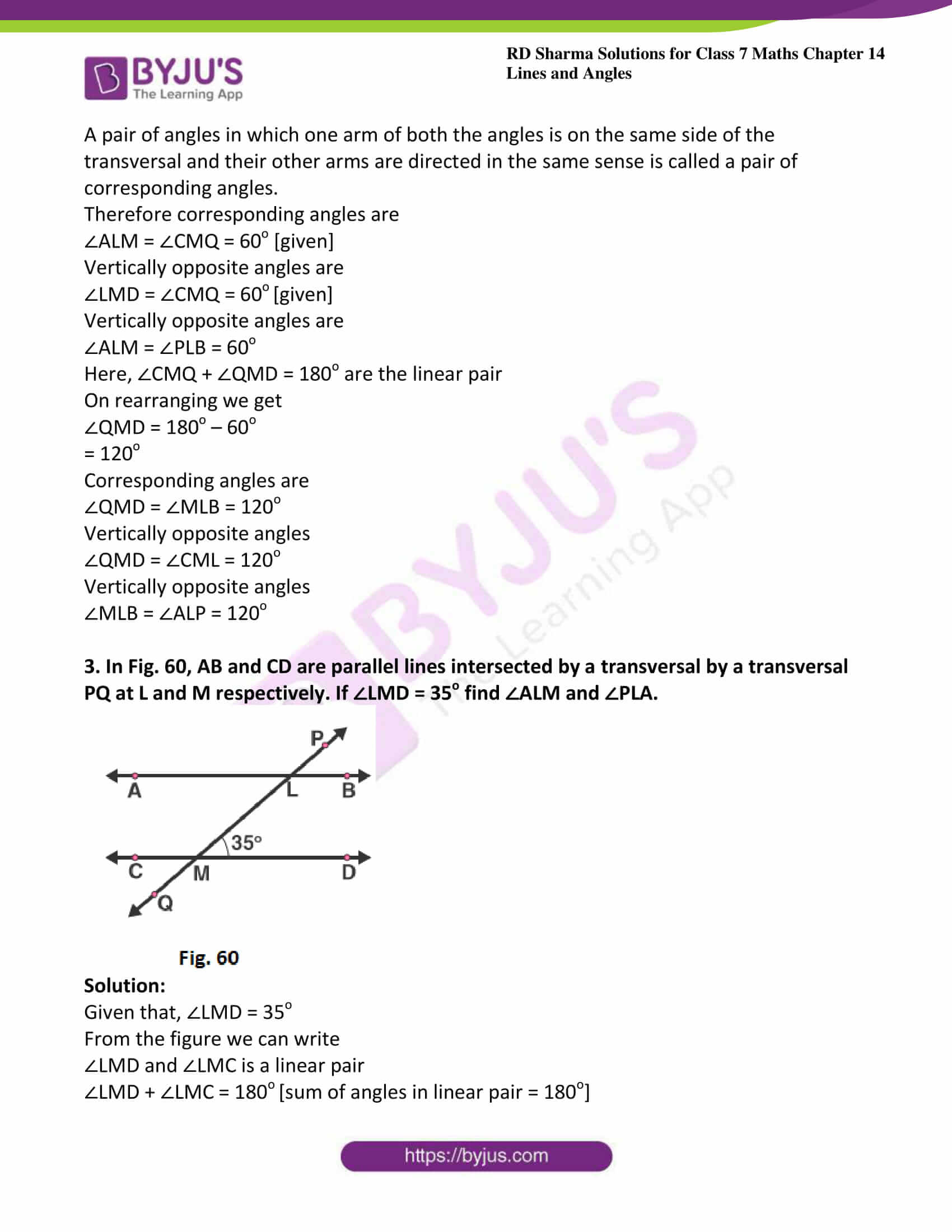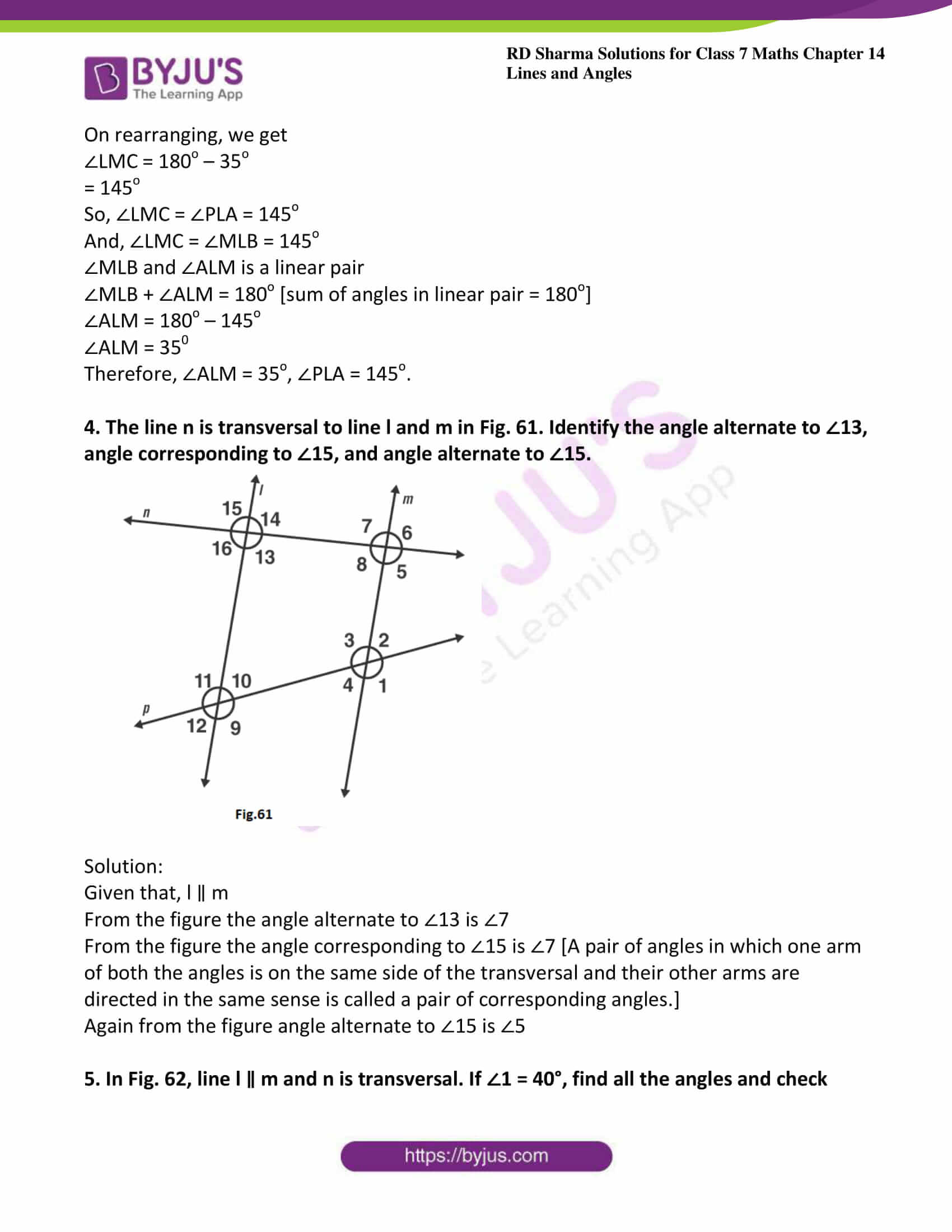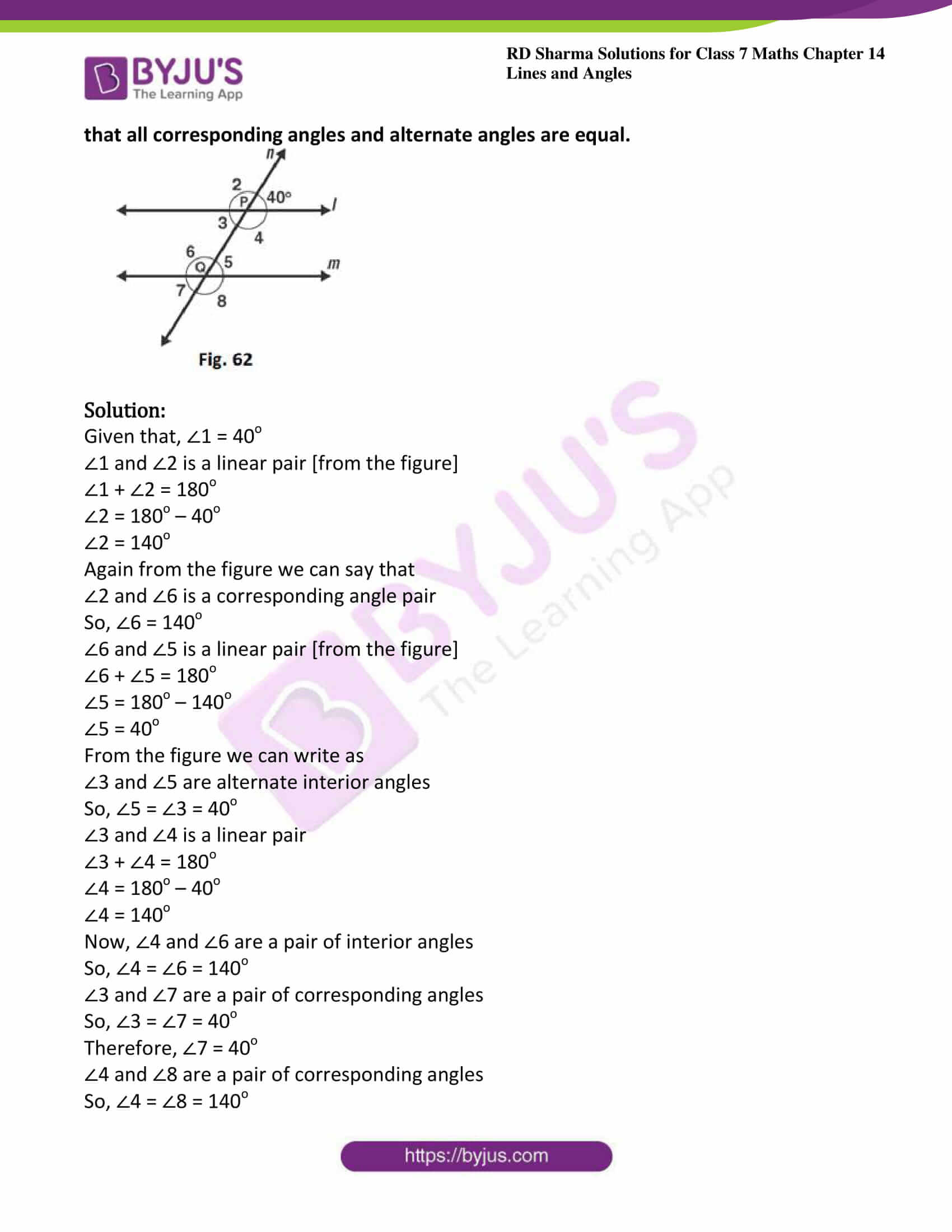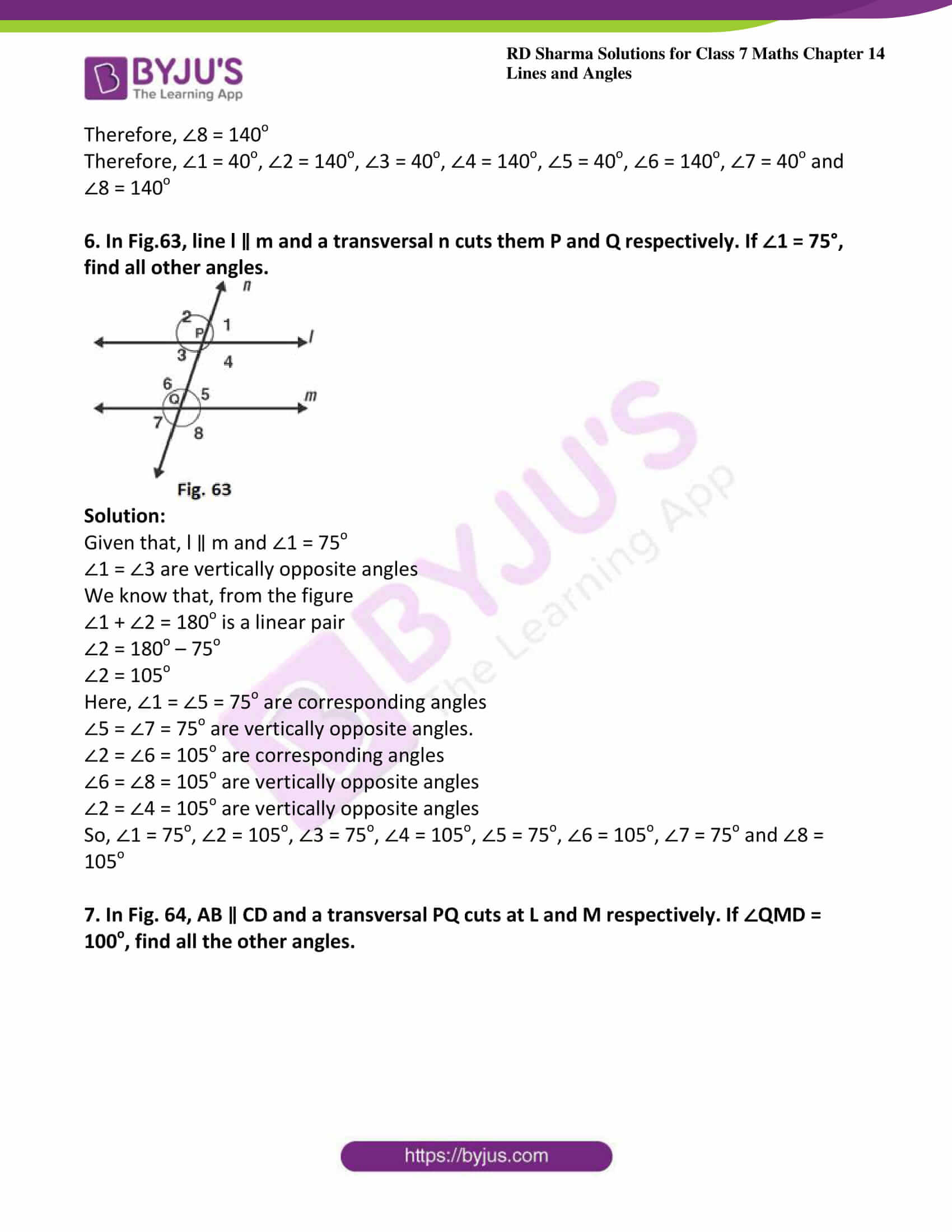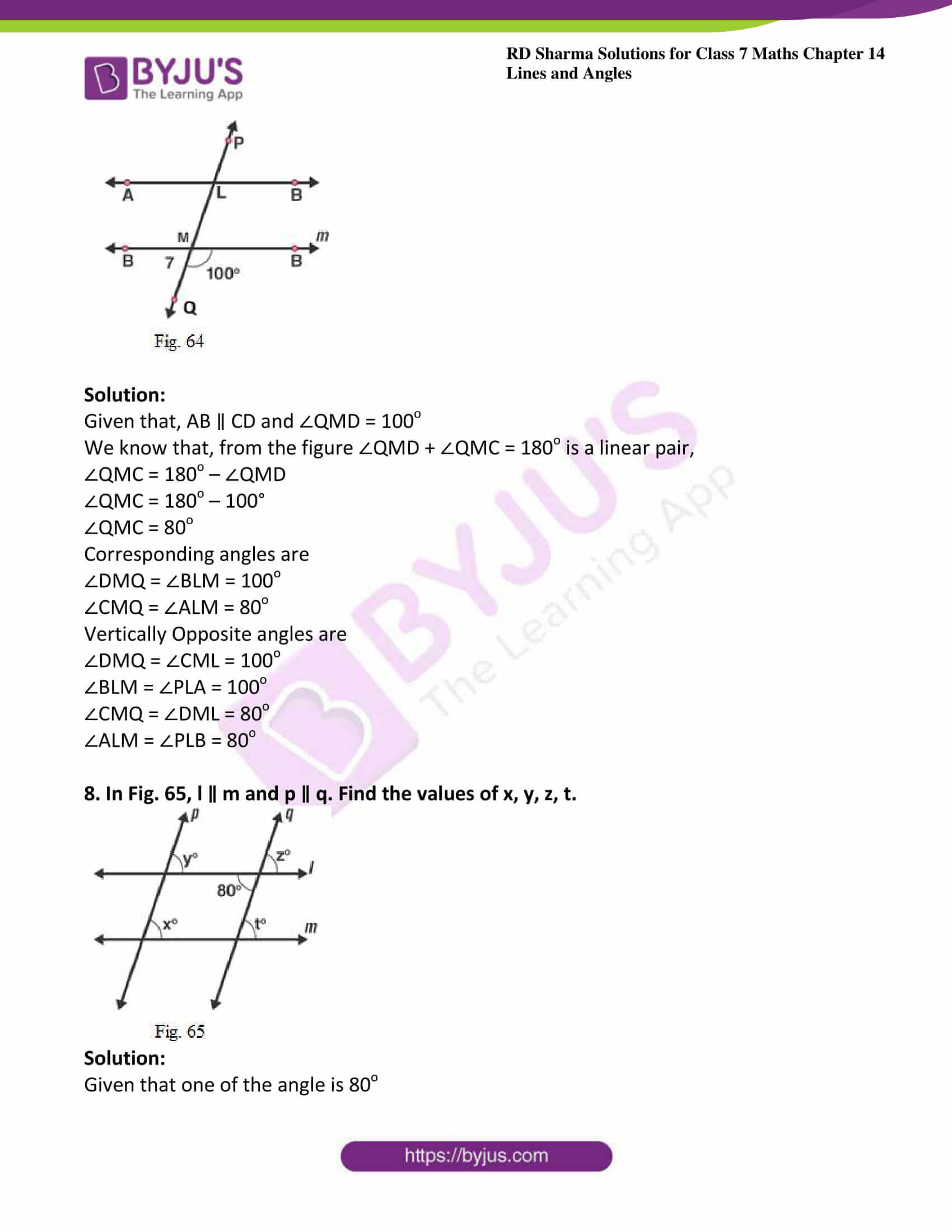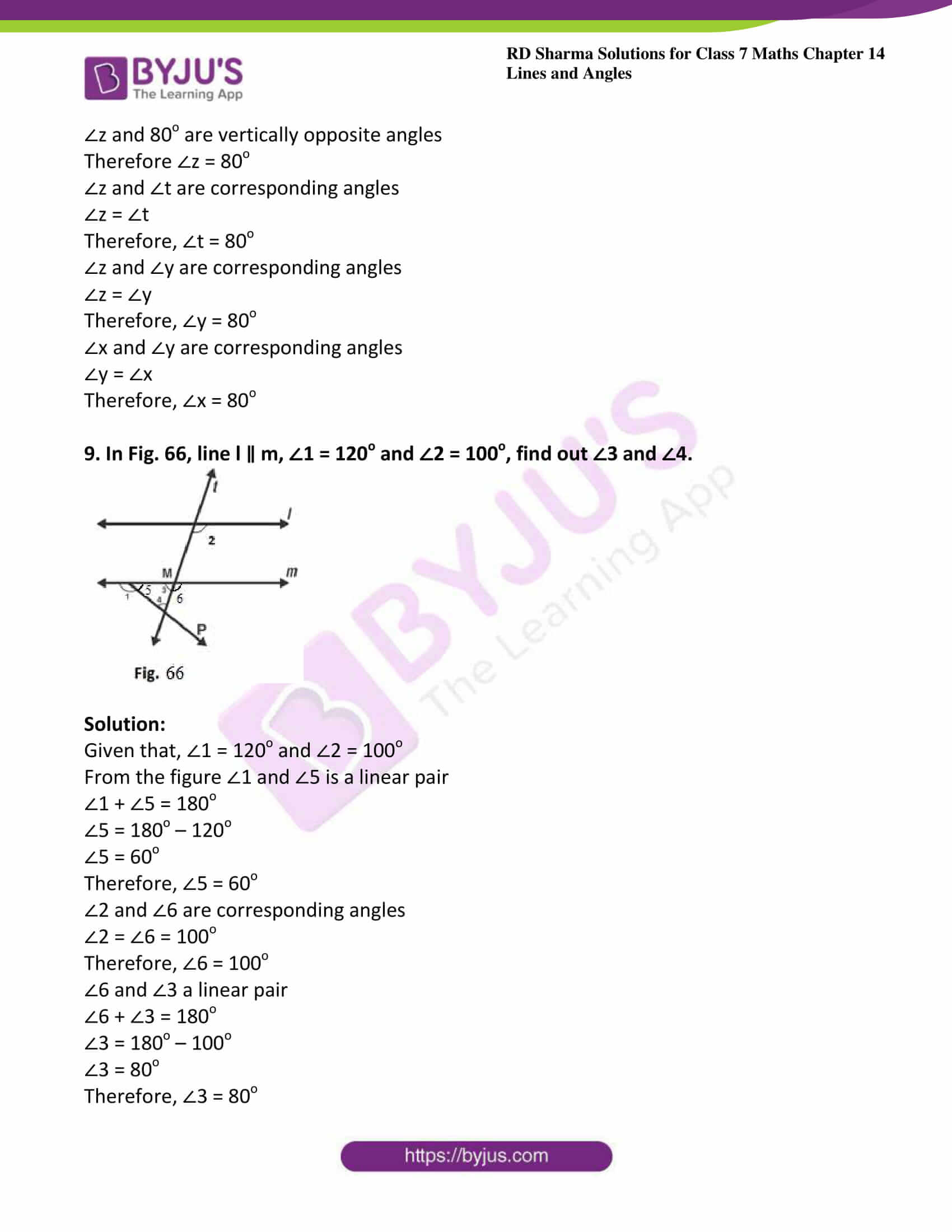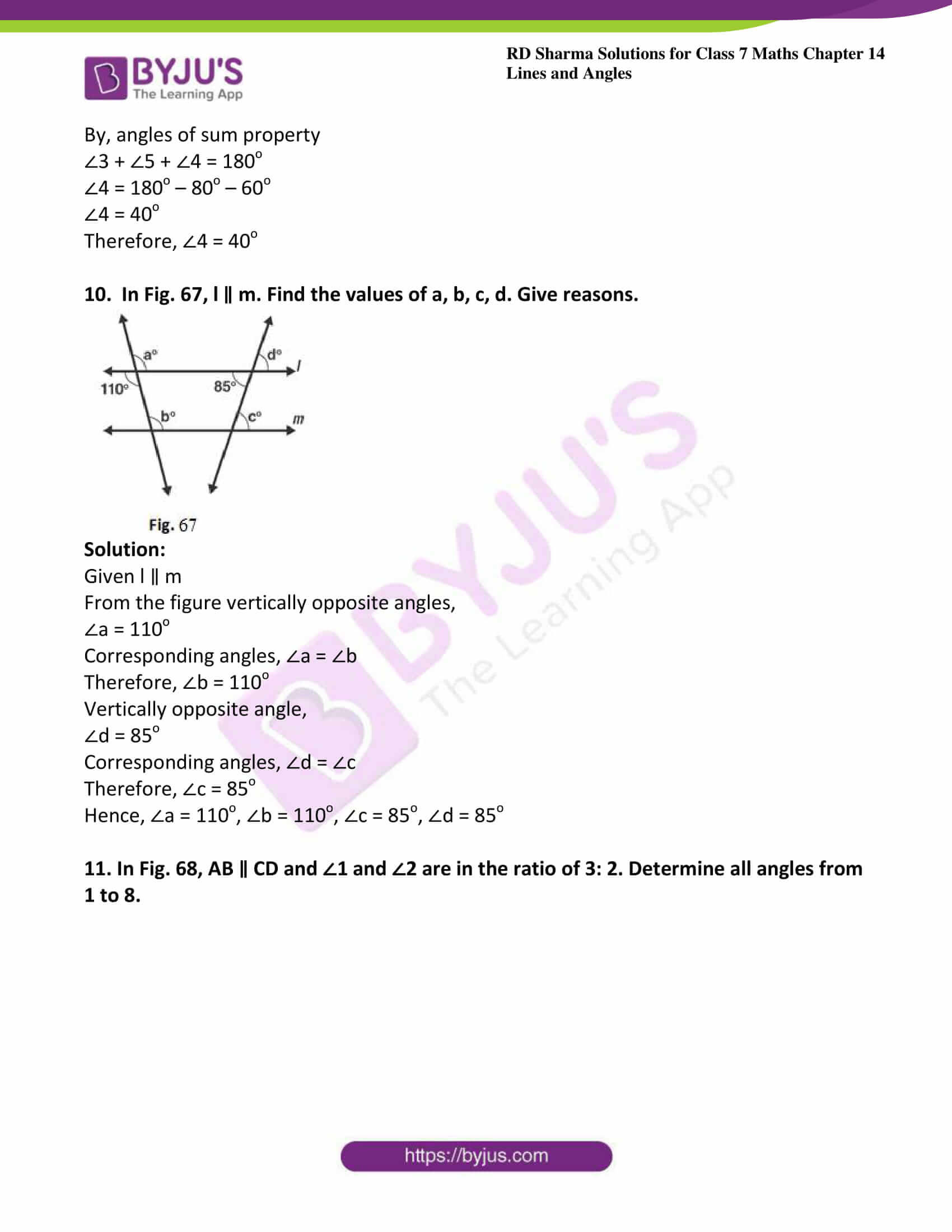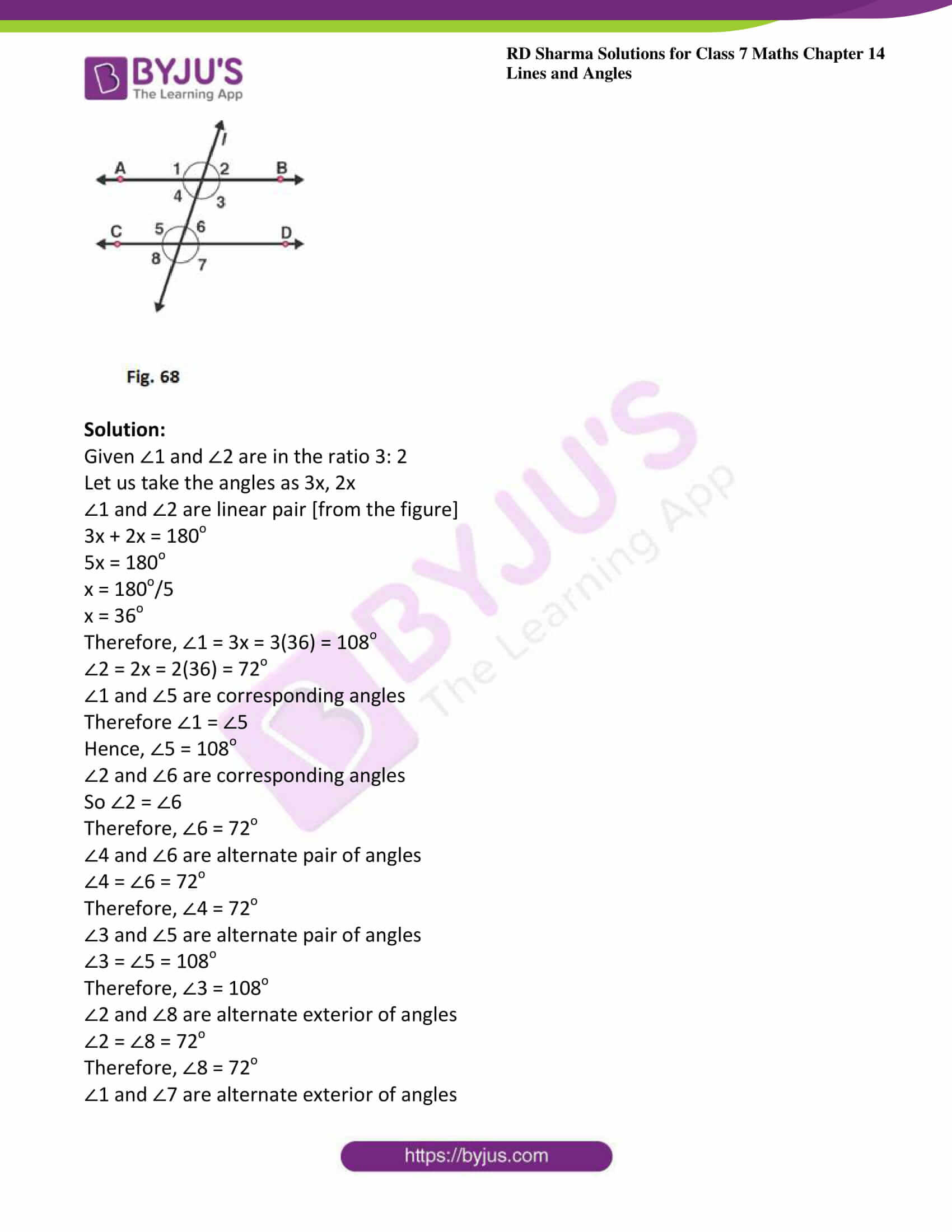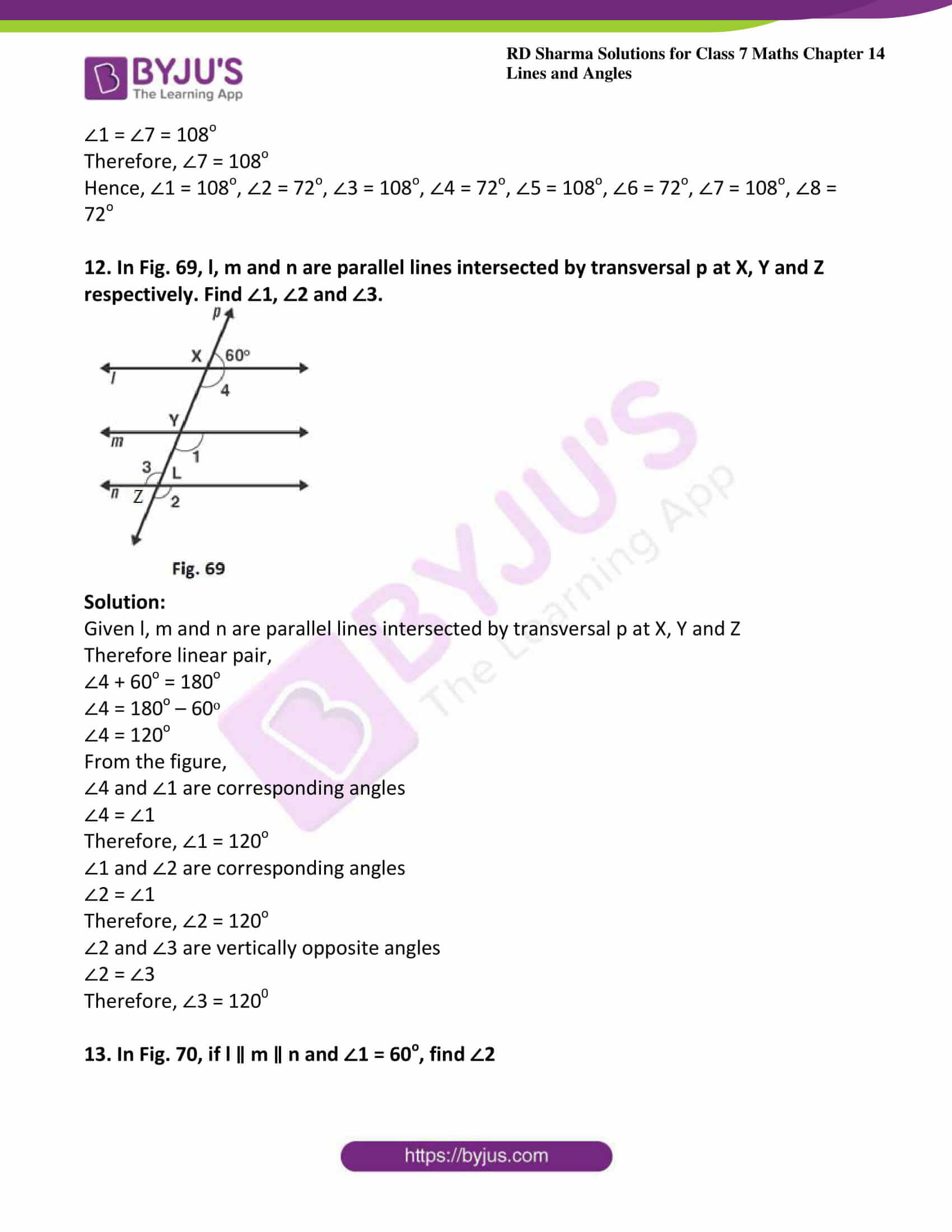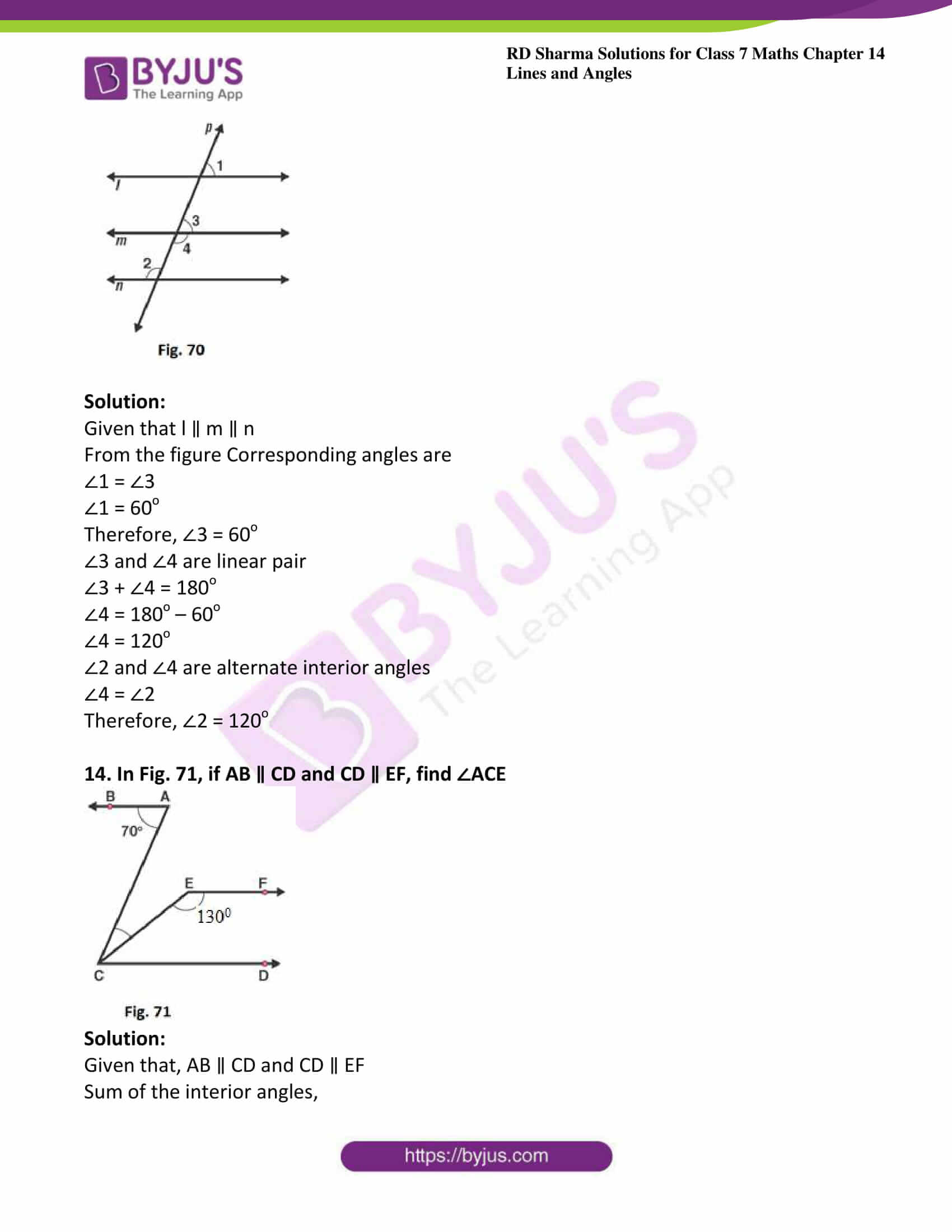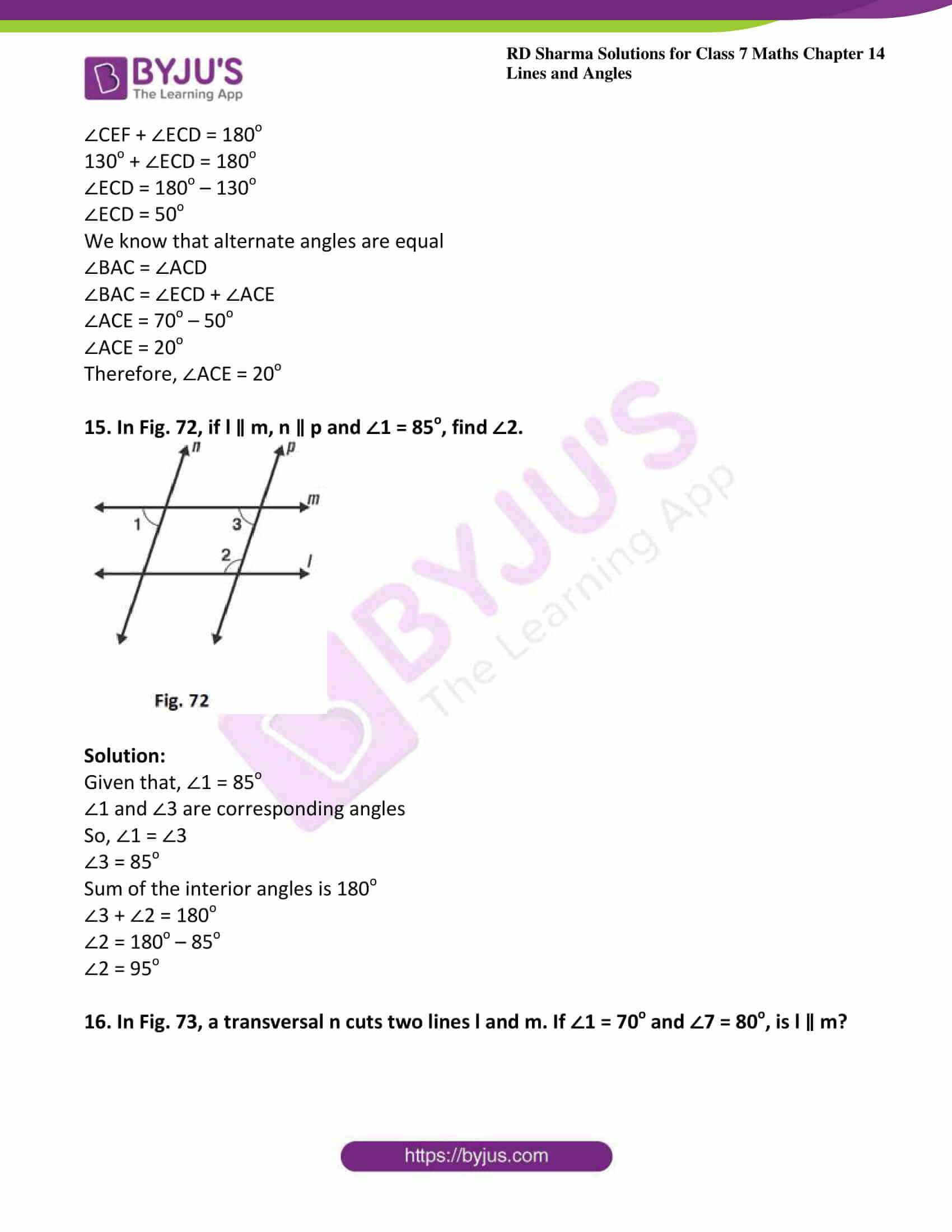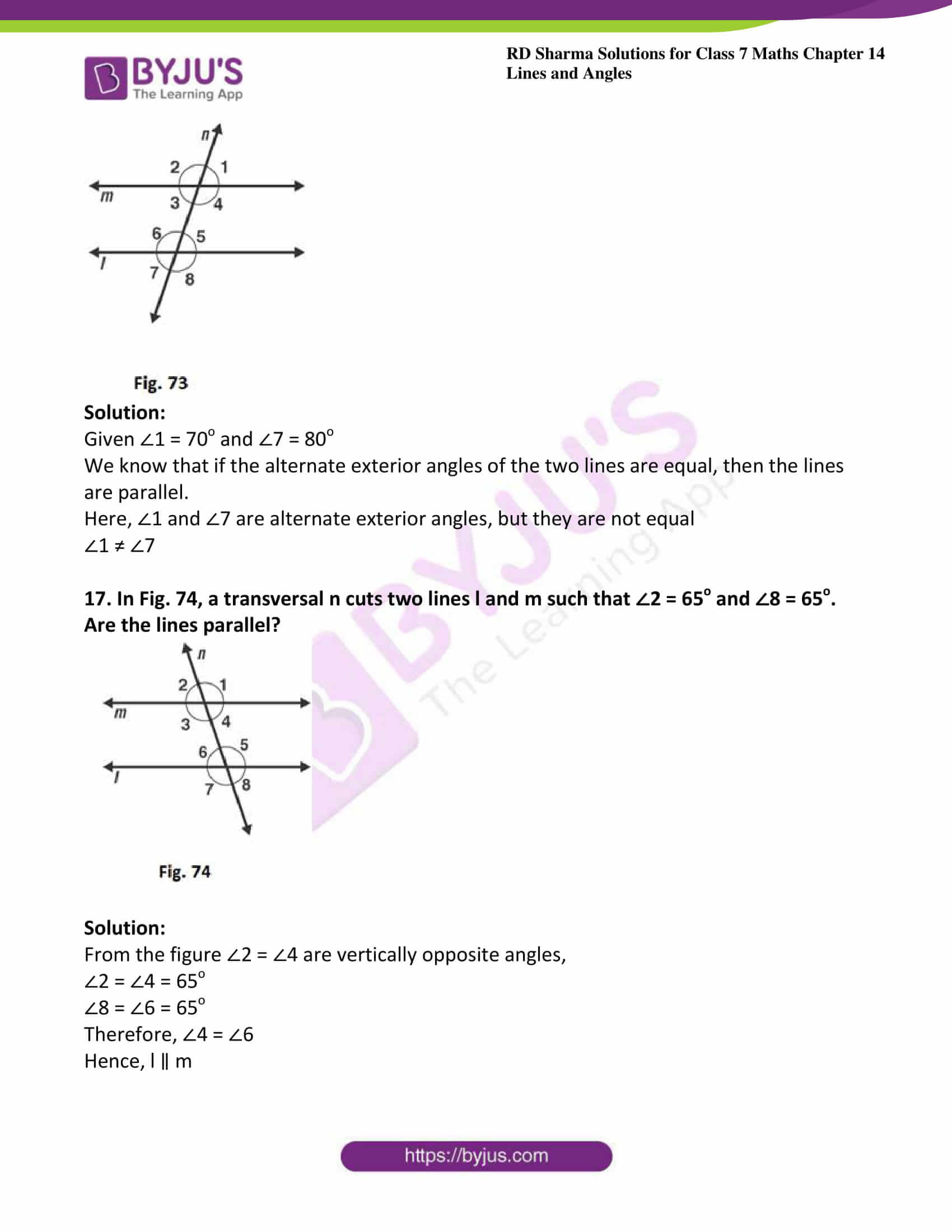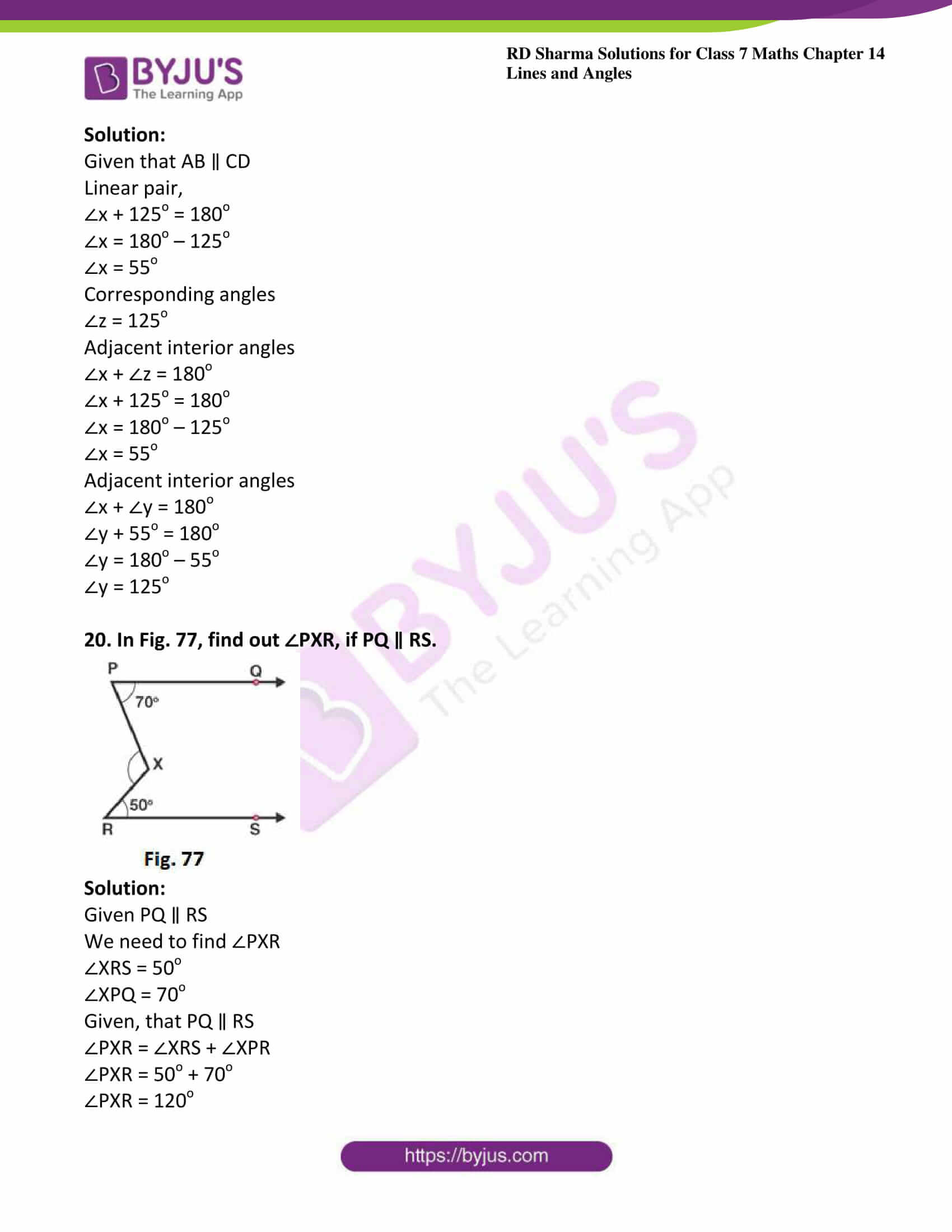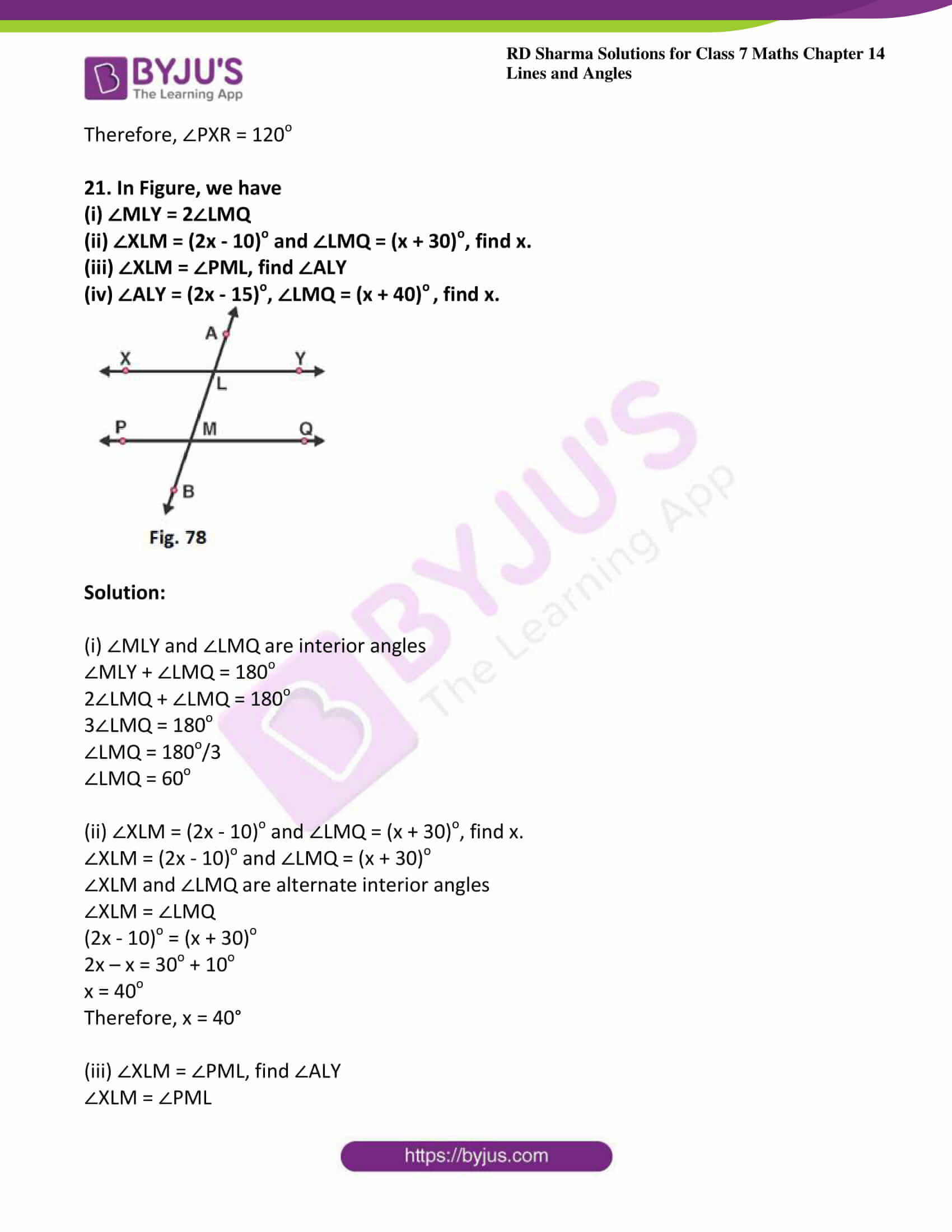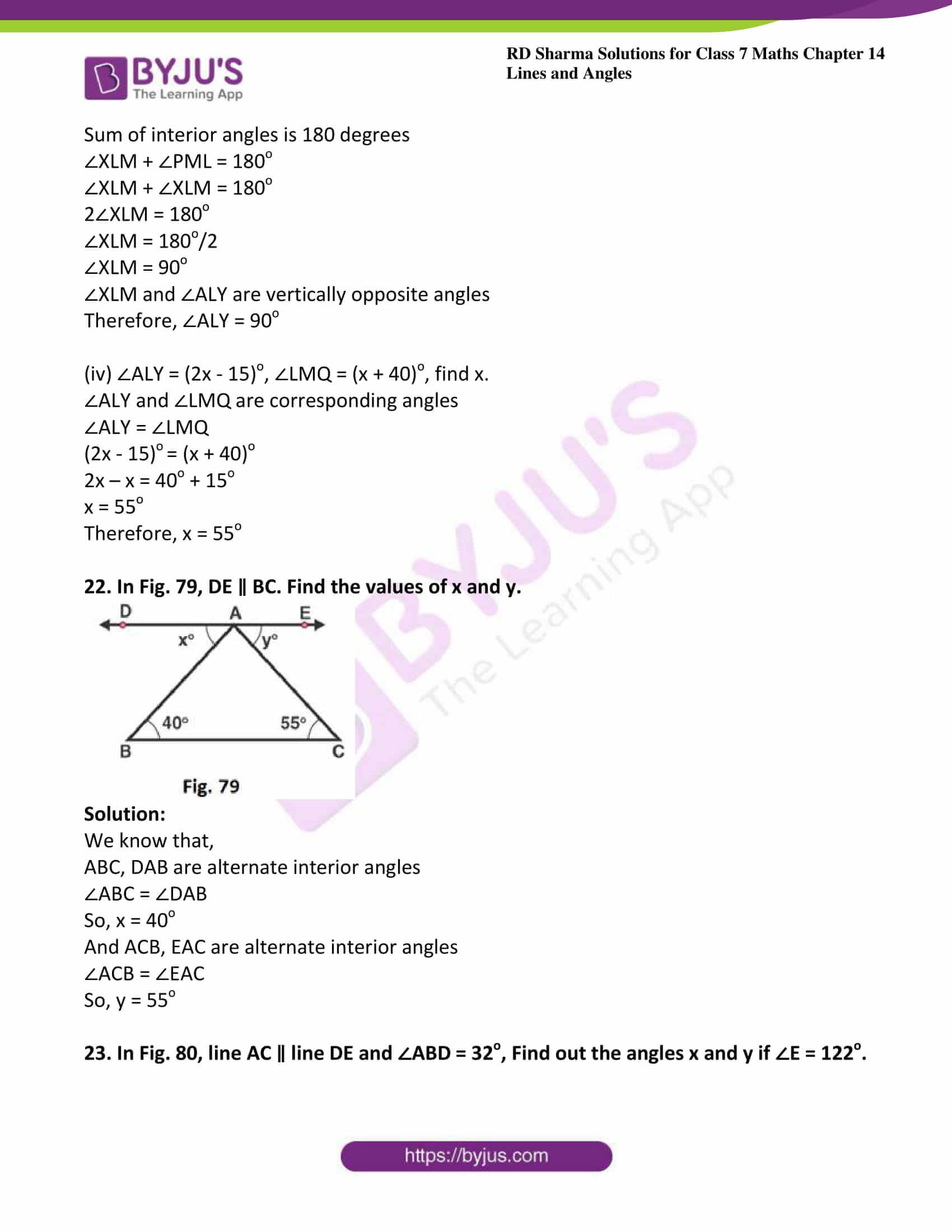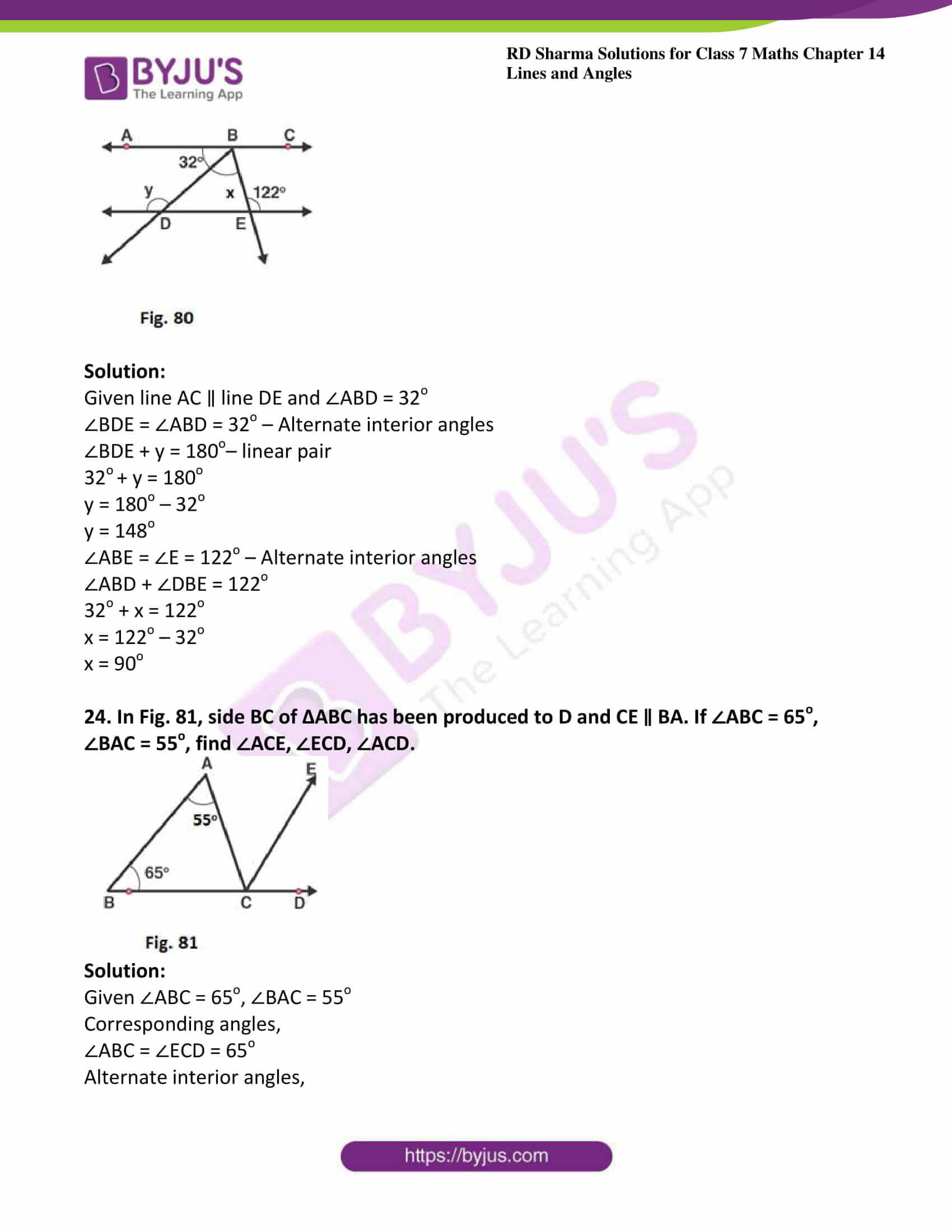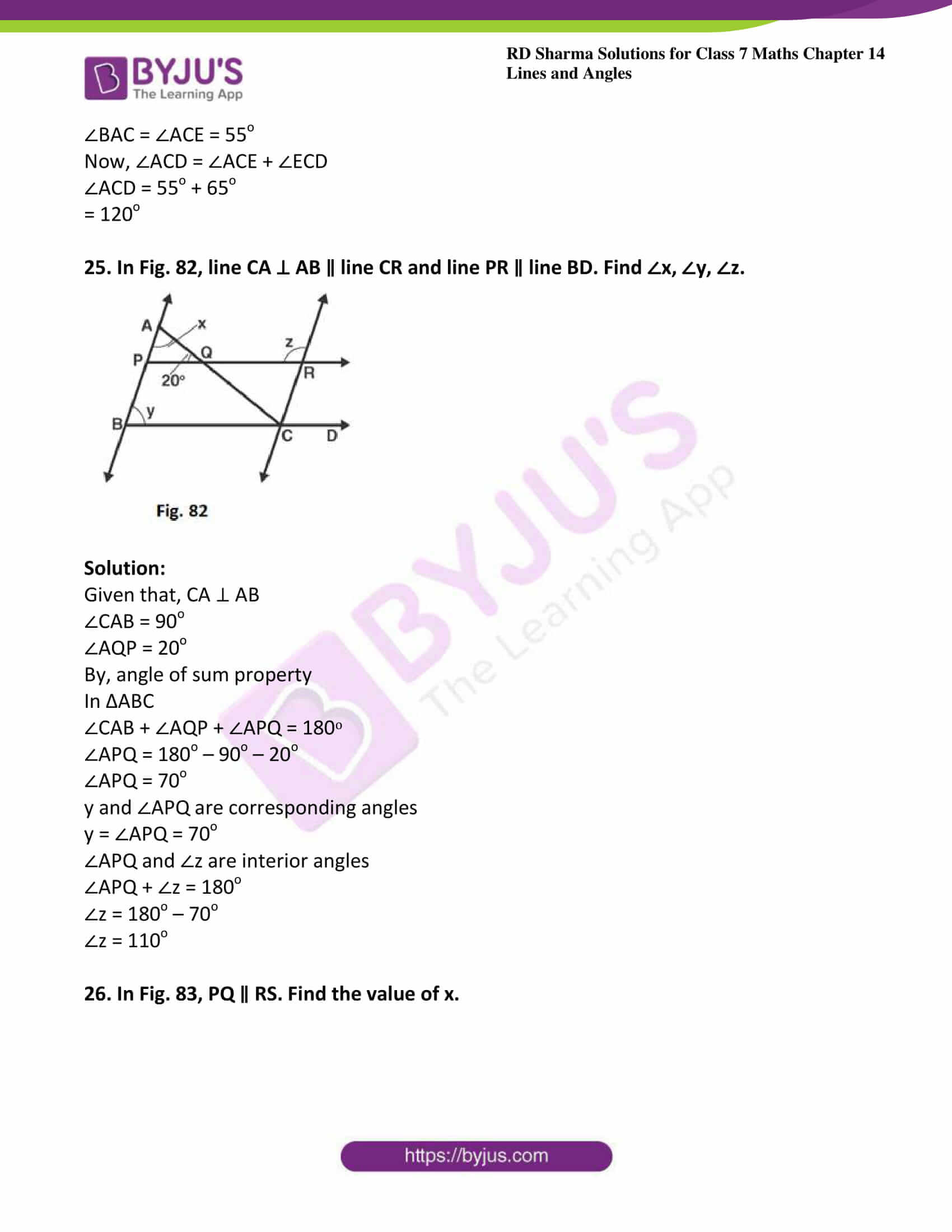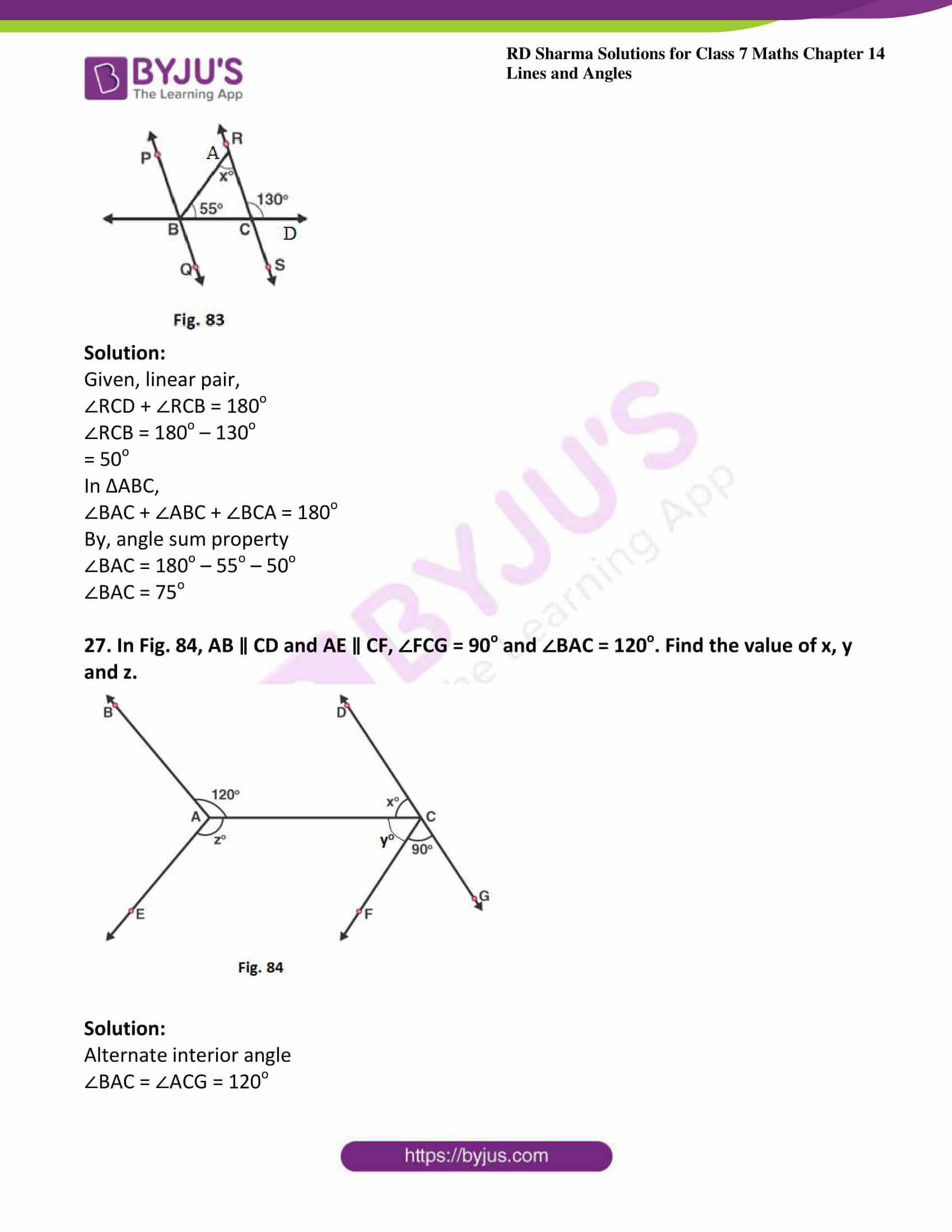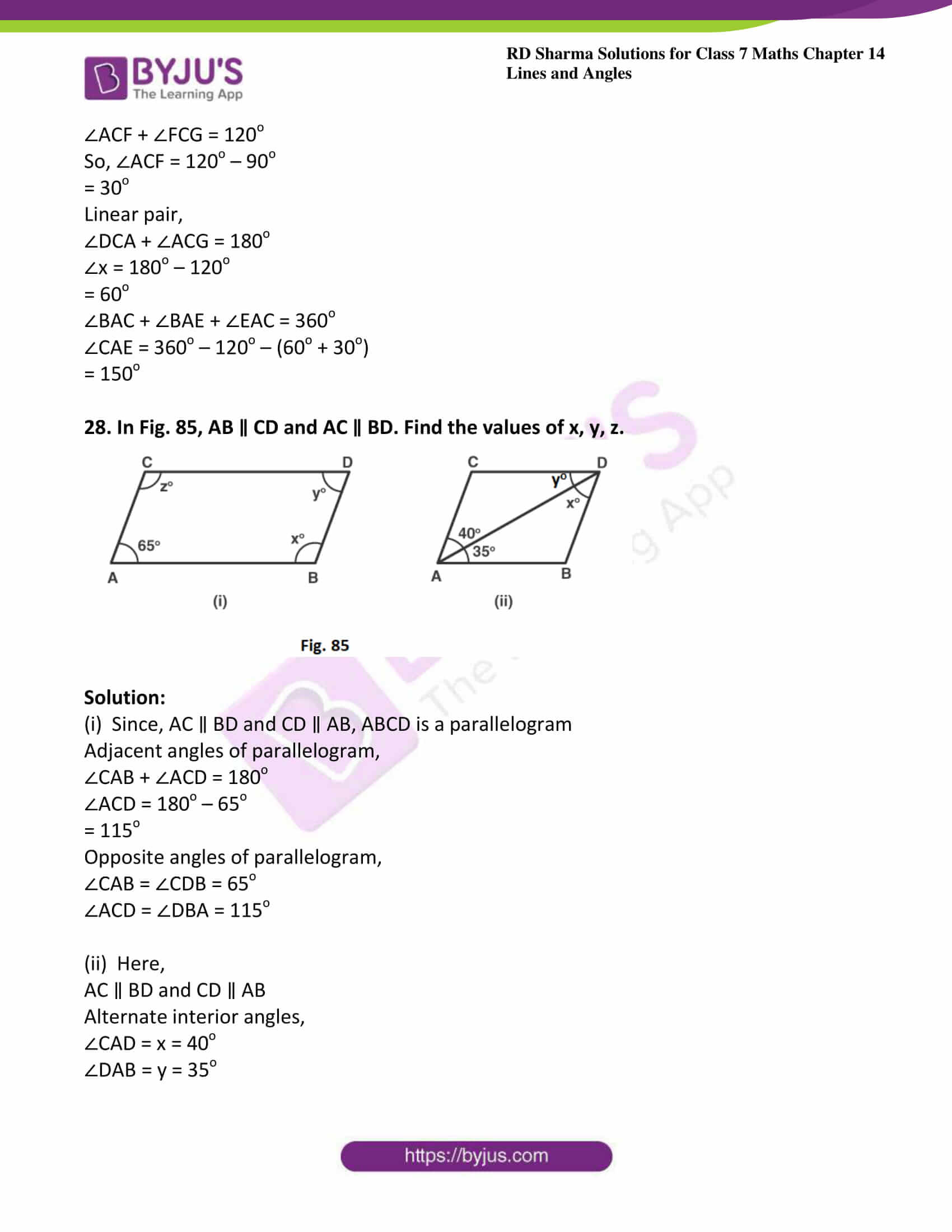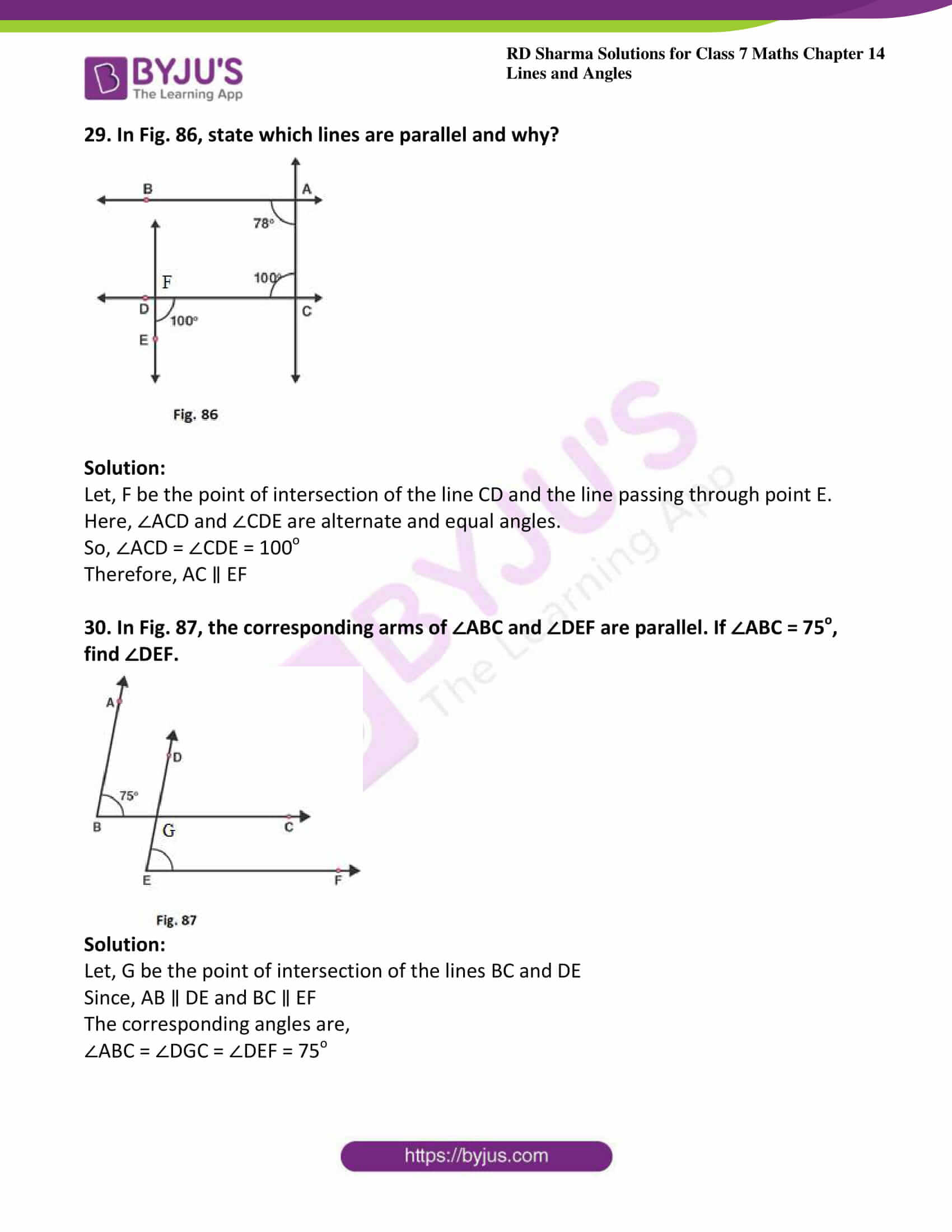### Access answers to Maths RD Sharma Solutions For Class 7 Chapter 14 – Lines And Angles Exercise 14.2

Exercise 14.2 Page No: 14.20

1. In Fig. 58, line n is a transversal to line l and m. Identify the following:

(i) Alternate and corresponding angles in Fig. 58 (i)

(ii) Angles alternate to ∠d and ∠g and angles corresponding to ∠f and ∠h in Fig. 58 (ii)

(iii) Angle alternate to ∠PQR, angle corresponding to ∠RQF and angle alternate to ∠PQE in Fig. 58 (iii)

(iv) Pairs of interior and exterior angles on the same side of the transversal in Fig. 58 (ii)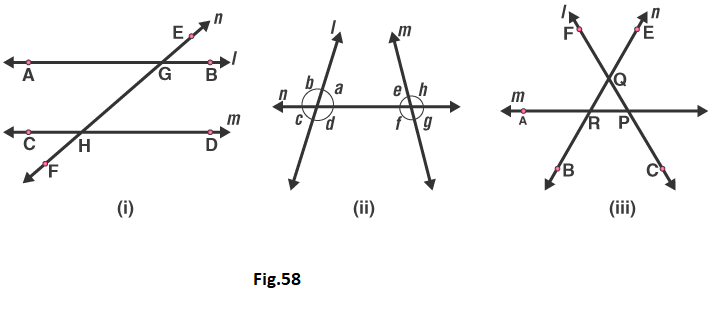Solution:

(i) A pair of angles in which one arm of both the angles is on the same side of the transversal and their other arms are directed in the same sense is called a pair of corresponding angles.

In Figure (i) Corresponding angles are

∠EGB and ∠GHD

∠HGB and ∠FHD

∠EGA and ∠GHC

∠AGH and ∠CHF

A pair of angles in which one arm of each of the angle is on opposite sides of the transversal and whose other arms include the one segment is called a pair of alternate angles.

The alternate angles are:

∠EGB and ∠CHF

∠HGB and ∠CHG

∠EGA and ∠FHD

∠AGH and ∠GHD

(ii) In Figure (ii)

The alternate angle to ∠d is ∠e.

The alternate angle to ∠g is ∠b.

The corresponding angle to ∠f is ∠c.

The corresponding angle to ∠h is ∠a.

(iii) In Figure (iii)

Angle alternate to ∠PQR is ∠QRA.

Angle corresponding to ∠RQF is ∠ARB.

Angle alternate to ∠POE is ∠ARB.

(iv) In Figure (ii)

Pair of interior angles are

∠a is ∠e.

∠d is ∠f.

Pair of exterior angles are

∠b is ∠h.

∠c is ∠g.

2. In Fig. 59, AB and CD are parallel lines intersected by a transversal PQ at L and M respectively, If ∠CMQ = 60o, find all other angles in the figure.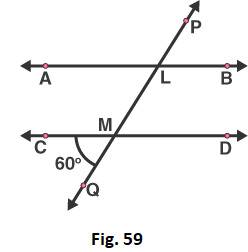Solution:

A pair of angles in which one arm of both the angles is on the same side of the transversal and their other arms are directed in the same sense is called a pair of corresponding angles.

Therefore corresponding angles are

∠ALM = ∠CMQ = 60o [given]

Vertically opposite angles are

∠LMD = ∠CMQ = 60o [given]

Vertically opposite angles are

∠ALM = ∠PLB = 60o

Here, ∠CMQ + ∠QMD = 180o are the linear pair

On rearranging we get

∠QMD = 180o – 60o

= 120o

Corresponding angles are

∠QMD = ∠MLB = 120o

Vertically opposite angles

∠QMD = ∠CML = 120o

Vertically opposite angles

∠MLB = ∠ALP = 120o

3. In Fig. 60, AB and CD are parallel lines intersected by a transversal by a transversal PQ at L and M respectively. If ∠LMD = 35o find ∠ALM and ∠PLA.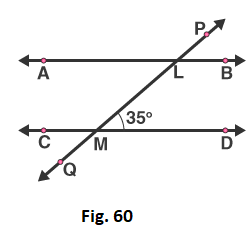Solution:

Given that, ∠LMD = 35o

From the figure we can write

∠LMD and ∠LMC is a linear pair

∠LMD + ∠LMC = 180o [sum of angles in linear pair = 180o]

On rearranging, we get

∠LMC = 180o – 35o

= 145o

So, ∠LMC = ∠PLA = 145o

And, ∠LMC = ∠MLB = 145o

∠MLB and ∠ALM is a linear pair

∠MLB + ∠ALM = 180o [sum of angles in linear pair = 180o]

∠ALM = 180o – 145o

∠ALM = 350

Therefore, ∠ALM = 35o, ∠PLA = 145o.

4. The line n is transversal to line l and m in Fig. 61. Identify the angle alternate to ∠13, angle corresponding to ∠15, and angle alternate to ∠15.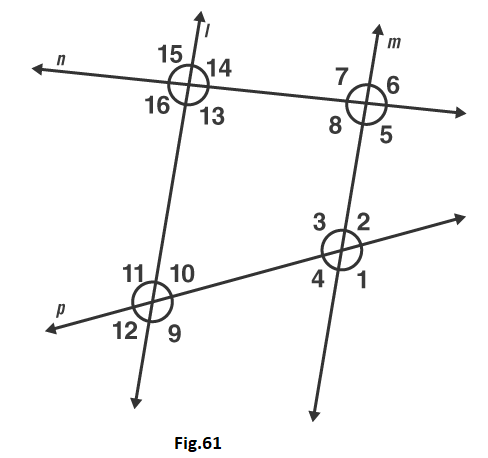Solution:

Given that, l ∥ m

From the figure the angle alternate to ∠13 is ∠7

From the figure the angle corresponding to ∠15 is ∠7 [A pair of angles in which one arm of both the angles is on the same side of the transversal and their other arms are directed in the same sense is called a pair of corresponding angles.]

Again from the figure angle alternate to ∠15 is ∠5

5. In Fig. 62, line l ∥ m and n is transversal. If ∠1 = 40°, find all the angles and check that all corresponding angles and alternate angles are equal.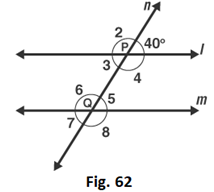Solution:

Given that, ∠1 = 40o

∠1 and ∠2 is a linear pair [from the figure]

∠1 + ∠2 = 180o

∠2 = 180o – 40o

∠2 = 140o

Again from the figure we can say that

∠2 and ∠6 is a corresponding angle pair

So, ∠6 = 140o

∠6 and ∠5 is a linear pair [from the figure]

∠6 + ∠5 = 180o

∠5 = 180o – 140o

∠5 = 40o

From the figure we can write as

∠3 and ∠5 are alternate interior angles

So, ∠5 = ∠3 = 40o

∠3 and ∠4 is a linear pair

∠3 + ∠4 = 180o

∠4 = 180o – 40o

∠4 = 140o

Now, ∠4 and ∠6 are a pair of interior angles

So, ∠4 = ∠6 = 140o

∠3 and ∠7 are a pair of corresponding angles

So, ∠3 = ∠7 = 40o

Therefore, ∠7 = 40o

∠4 and ∠8 are a pair of corresponding angles

So, ∠4 = ∠8 = 140o

Therefore, ∠8 = 140o

Therefore, ∠1 = 40o, ∠2 = 140o, ∠3 = 40o, ∠4 = 140o, ∠5 = 40o, ∠6 = 140o, ∠7 = 40o and ∠8 = 140o

6. In Fig.63, line l ∥ m and a transversal n cuts them P and Q respectively. If ∠1 = 75°, find all other angles.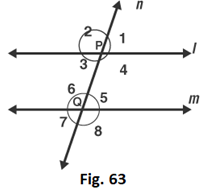Solution:

Given that, l ∥ m and ∠1 = 75o

∠1 = ∠3 are vertically opposite angles

We know that, from the figure

∠1 + ∠2 = 180o is a linear pair

∠2 = 180o – 75o

∠2 = 105o

Here, ∠1 = ∠5 = 75o are corresponding angles

∠5 = ∠7 = 75o are vertically opposite angles.

∠2 = ∠6 = 105o are corresponding angles

∠6 = ∠8 = 105o are vertically opposite angles

∠2 = ∠4 = 105o are vertically opposite angles

So, ∠1 = 75o, ∠2 = 105o, ∠3 = 75o, ∠4 = 105o, ∠5 = 75o, ∠6 = 105o, ∠7 = 75o and ∠8 = 105o

7. In Fig. 64, AB ∥ CD and a transversal PQ cuts at L and M respectively. If ∠QMD = 100o, find all the other angles.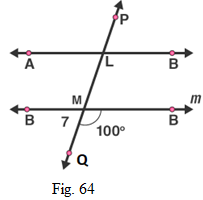Solution:

Given that, AB ∥ CD and ∠QMD = 100o

We know that, from the figure ∠QMD + ∠QMC = 180o is a linear pair,

∠QMC = 180o – ∠QMD

∠QMC = 180o – 100°

∠QMC = 80o

Corresponding angles are

∠DMQ = ∠BLM = 100o

∠CMQ = ∠ALM = 80o

Vertically Opposite angles are

∠DMQ = ∠CML = 100o

∠BLM = ∠PLA = 100o

∠CMQ = ∠DML = 80o

∠ALM = ∠PLB = 80o

8. In Fig. 65, l ∥ m and p ∥ q. Find the values of x, y, z, t.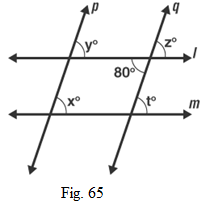Solution:

Given that one of the angle is 80o

∠z and 80o are vertically opposite angles

Therefore ∠z = 80o

∠z and ∠t are corresponding angles

∠z = ∠t

Therefore, ∠t = 80o

∠z and ∠y are corresponding angles

∠z = ∠y

Therefore, ∠y = 80o

∠x and ∠y are corresponding angles

∠y = ∠x

Therefore, ∠x = 80o

9. In Fig. 66, line l ∥ m, ∠1 = 120o and ∠2 = 100o, find out ∠3 and ∠4.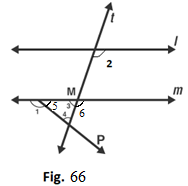Solution:

Given that, ∠1 = 120o and ∠2 = 100o

From the figure ∠1 and ∠5 is a linear pair

∠1 + ∠5 = 180o

∠5 = 180o – 120o

∠5 = 60o

Therefore, ∠5 = 60o

∠2 and ∠6 are corresponding angles

∠2 = ∠6 = 100o

Therefore, ∠6 = 100o

∠6 and ∠3 a linear pair

∠6 + ∠3 = 180o

∠3 = 180o – 100o

∠3 = 80o

Therefore, ∠3 = 80o

By, angles of sum property

∠3 + ∠5 + ∠4 = 180o

∠4 = 180o – 80o – 60o

∠4 = 40o

Therefore, ∠4 = 40o

10.  In Fig. 67, l ∥ m. Find the values of a, b, c, d. Give reasons.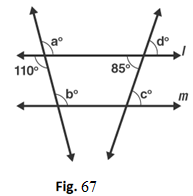Solution:

Given l ∥ m

From the figure vertically opposite angles,

∠a = 110o

Corresponding angles, ∠a = ∠b

Therefore, ∠b = 110o

Vertically opposite angle,

∠d = 85o

Corresponding angles, ∠d = ∠c

Therefore, ∠c = 85o

Hence, ∠a = 110o, ∠b = 110o, ∠c = 85o, ∠d = 85o

11. In Fig. 68, AB ∥ CD and ∠1 and ∠2 are in the ratio of 3: 2. Determine all angles from 1 to 8.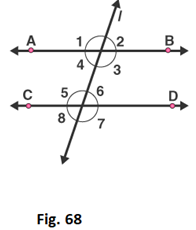Solution:

Given ∠1 and ∠2 are in the ratio 3: 2

Let us take the angles as 3x, 2x

∠1 and ∠2 are linear pair [from the figure]

3x + 2x = 180o

5x = 180o

x = 180o/5

x = 36o

Therefore, ∠1 = 3x = 3(36) = 108o

∠2 = 2x = 2(36) = 72o

∠1 and ∠5 are corresponding angles

Therefore ∠1 = ∠5

Hence, ∠5 = 108o

∠2 and ∠6 are corresponding angles

So ∠2 = ∠6

Therefore, ∠6 = 72o

∠4 and ∠6 are alternate pair of angles

∠4 = ∠6 = 72o

Therefore, ∠4 = 72o

∠3 and ∠5 are alternate pair of angles

∠3 = ∠5 = 108o

Therefore, ∠3 = 108o

∠2 and ∠8 are alternate exterior of angles

∠2 = ∠8 = 72o

Therefore, ∠8 = 72o

∠1 and ∠7 are alternate exterior of angles

∠1 = ∠7 = 108o

Therefore, ∠7 = 108o

Hence, ∠1 = 108o, ∠2 = 72o, ∠3 = 108o, ∠4 = 72o, ∠5 = 108o, ∠6 = 72o, ∠7 = 108o, ∠8 = 72o

12. In Fig. 69, l, m and n are parallel lines intersected by transversal p at X, Y and Z respectively. Find ∠1, ∠2 and ∠3.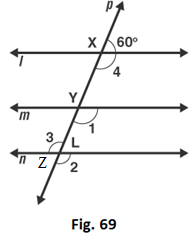Solution:

Given l, m and n are parallel lines intersected by transversal p at X, Y and Z

Therefore linear pair,

∠4 + 60o = 180o

∠4 = 180o – 60o

∠4 = 120o

From the figure,

∠4 and ∠1 are corresponding angles

∠4 = ∠1

Therefore, ∠1 = 120o

∠1 and ∠2 are corresponding angles

∠2 = ∠1

Therefore, ∠2 = 120o

∠2 and ∠3 are vertically opposite angles

∠2 = ∠3

Therefore, ∠3 = 1200

13. In Fig. 70, if l ∥ m ∥ n and ∠1 = 60o, find ∠2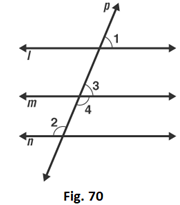Solution:

Given that l ∥ m ∥ n

From the figure Corresponding angles are

∠1 = ∠3

∠1 = 60o

Therefore, ∠3 = 60o

∠3 and ∠4 are linear pair

∠3 + ∠4 = 180o

∠4 = 180o – 60o

∠4 = 120o

∠2 and ∠4 are alternate interior angles

∠4 = ∠2

Therefore, ∠2 = 120o

14. In Fig. 71, if AB ∥ CD and CD ∥ EF, find ∠ACE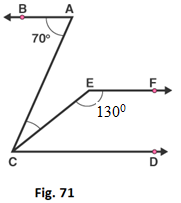Solution:

Given that, AB ∥ CD and CD ∥ EF

Sum of the interior angles,

∠CEF + ∠ECD = 180o

130o + ∠ECD = 180o

∠ECD = 180o – 130o

∠ECD = 50o

We know that alternate angles are equal

∠BAC = ∠ACD

∠BAC = ∠ECD + ∠ACE

∠ACE = 70o – 50o

∠ACE = 20o

Therefore, ∠ACE = 20o

15. In Fig. 72, if l ∥ m, n ∥ p and ∠1 = 85o, find ∠2.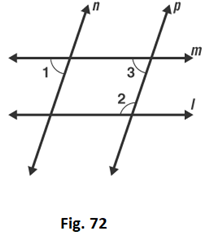Solution:

Given that, ∠1 = 85o

∠1 and ∠3 are corresponding angles

So, ∠1 = ∠3

∠3 = 85o

Sum of the interior angles is 180o

∠3 + ∠2 = 180o

∠2 = 180o – 85o

∠2 = 95o

16. In Fig. 73, a transversal n cuts two lines l and m. If ∠1 = 70o and ∠7 = 80o, is l ∥ m?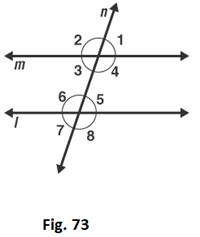Solution:

Given ∠1 = 70o and ∠7 = 80o

We know that if the alternate exterior angles of the two lines are equal, then the lines are parallel.

Here, ∠1 and ∠7 are alternate exterior angles, but they are not equal

∠1 ≠ ∠7

17. In Fig. 74, a transversal n cuts two lines l and m such that ∠2 = 65o and ∠8 = 65o. Are the lines parallel?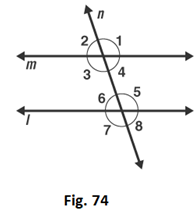Solution:

From the figure ∠2 = ∠4 are vertically opposite angles,

∠2 = ∠4 = 65o

∠8 = ∠6 = 65o

Therefore, ∠4 = ∠6

Hence, l ∥ m

18. In Fig. 75, Show that AB ∥ EF.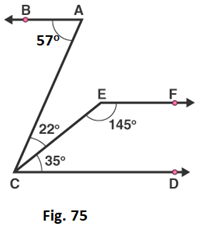Solution:

We know that,

∠ACD = ∠ACE + ∠ECD

∠ACD = 22o + 35o

∠ACD = 57o = ∠BAC

Thus, lines BA and CD are intersected by the line AC such that, ∠ACD = ∠BAC

So, the alternate angles are equal

Therefore, AB ∥ CD ……1

Now,

∠ECD + ∠CEF = 35o + 145o = 180o

This, shows that sum of the angles of the interior angles on the same side of the transversal CE is 180o

So, they are supplementary angles

Therefore, EF ∥ CD …….2

From equation 1 and 2

We conclude that, AB ∥ EF

19. In Fig. 76, AB ∥ CD. Find the values of x, y, z.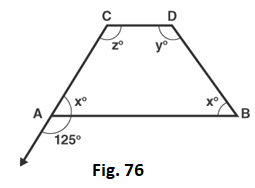Solution:

Given that AB ∥ CD

Linear pair,

∠x + 125o = 180o

∠x = 180o – 125o

∠x = 55o

Corresponding angles

∠z = 125o

∠x + ∠z = 180o

∠x + 125o = 180o

∠x = 180o – 125o

∠x = 55o

∠x + ∠y = 180o

∠y + 55o = 180o

∠y = 180o – 55o

∠y = 125o

20. In Fig. 77, find out ∠PXR, if PQ ∥ RS.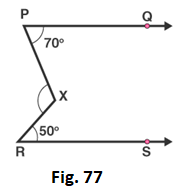Solution:

Given PQ ∥ RS

We need to find ∠PXR

∠XRS = 50o

∠XPQ = 70o

Given, that PQ ∥ RS

∠PXR = ∠XRS + ∠XPR

∠PXR = 50o + 70o

∠PXR = 120o

Therefore, ∠PXR = 120o

21. In Figure, we have

(i) ∠MLY = 2∠LMQ

(ii) ∠XLM = (2x – 10)o and ∠LMQ = (x + 30)o, find x.

(iii) ∠XLM = ∠PML, find ∠ALY

(iv) ∠ALY = (2x – 15)o, ∠LMQ = (x + 40)o , find x.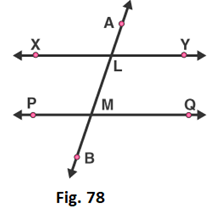Solution:

(i) ∠MLY and ∠LMQ are interior angles

∠MLY + ∠LMQ = 180o

2∠LMQ + ∠LMQ = 180o

3∠LMQ = 180o

∠LMQ = 180o/3

∠LMQ = 60o

(ii) ∠XLM = (2x – 10)o and ∠LMQ = (x + 30)o, find x.

∠XLM = (2x – 10)o and ∠LMQ = (x + 30)o

∠XLM and ∠LMQ are alternate interior angles

∠XLM = ∠LMQ

(2x – 10)o = (x + 30)o

2x – x = 30o + 10o

x = 40o

Therefore, x = 40°

(iii) ∠XLM = ∠PML, find ∠ALY

∠XLM = ∠PML

Sum of interior angles is 180 degrees

∠XLM + ∠PML = 180o

∠XLM + ∠XLM = 180o

2∠XLM = 180o

∠XLM = 180o/2

∠XLM = 90o

∠XLM and ∠ALY are vertically opposite angles

Therefore, ∠ALY = 90o

(iv) ∠ALY = (2x – 15)o, ∠LMQ = (x + 40)o, find x.

∠ALY and ∠LMQ are corresponding angles

∠ALY = ∠LMQ

(2x – 15)o = (x + 40)o

2x – x = 40o + 15o

x = 55o

Therefore, x = 55o

22. In Fig. 79, DE ∥ BC. Find the values of x and y.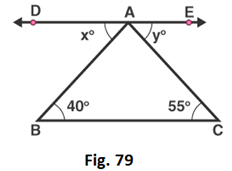Solution:

We know that,

ABC, DAB are alternate interior angles

∠ABC = ∠DAB

So, x = 40o

And ACB, EAC are alternate interior angles

∠ACB = ∠EAC

So, y = 55o

23. In Fig. 80, line AC ∥ line DE and ∠ABD = 32o, Find out the angles x and y if ∠E = 122o.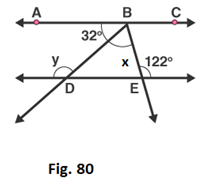Solution:

Given line AC ∥ line DE and ∠ABD = 32o

∠BDE = ∠ABD = 32o – Alternate interior angles

∠BDE + y = 180o– linear pair

32o + y = 180o

y = 180o – 32o

y = 148o

∠ABE = ∠E = 122o – Alternate interior angles

∠ABD + ∠DBE = 122o

32o + x = 122o

x = 122o – 32o

x = 90o

24. In Fig. 81, side BC of ΔABC has been produced to D and CE ∥ BA. If ∠ABC = 65o, ∠BAC = 55o, find ∠ACE, ∠ECD, ∠ACD.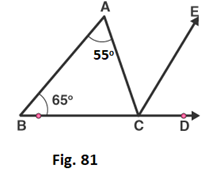Solution:

Given ∠ABC = 65o, ∠BAC = 55o

Corresponding angles,

∠ABC = ∠ECD = 65o

Alternate interior angles,

∠BAC = ∠ACE = 55o

Now, ∠ACD = ∠ACE + ∠ECD

∠ACD = 55o + 65o

= 120o

25. In Fig. 82, line CA ⊥ AB ∥ line CR and line PR ∥ line BD. Find ∠x, ∠y, ∠z.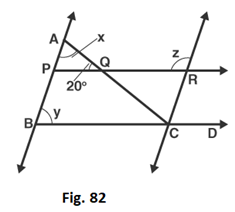Solution:

Given that, CA ⊥ AB

∠CAB = 90o

∠AQP = 20o

By, angle of sum property

In ΔABC

∠CAB + ∠AQP + ∠APQ = 180o

∠APQ = 180o – 90o – 20o

∠APQ = 70o

y and ∠APQ are corresponding angles

y = ∠APQ = 70o

∠APQ and ∠z are interior angles

∠APQ + ∠z = 180o

∠z = 180o – 70o

∠z = 110o

26. In Fig. 83, PQ ∥ RS. Find the value of x.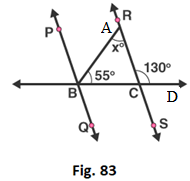Solution:

Given, linear pair,

∠RCD + ∠RCB = 180o

∠RCB = 180o – 130o

= 50o

In ΔABC,

∠BAC + ∠ABC + ∠BCA = 180o

By, angle sum property

∠BAC = 180o – 55o – 50o

∠BAC = 75o

27. In Fig. 84, AB ∥ CD and AE ∥ CF, ∠FCG = 90o and ∠BAC = 120o. Find the value of x, y and z.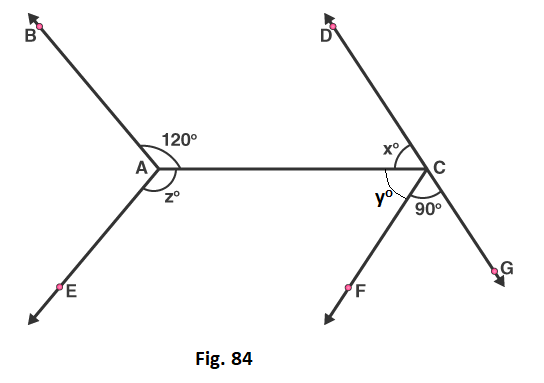Solution:

Alternate interior angle

∠BAC = ∠ACG = 120o

∠ACF + ∠FCG = 120o

So, ∠ACF = 120o – 90o

= 30o

Linear pair,

∠DCA + ∠ACG = 180o

∠x = 180o – 120o

= 60o

∠BAC + ∠BAE + ∠EAC = 360o

∠CAE = 360o – 120o – (60o + 30o)

= 150o

28. In Fig. 85, AB ∥ CD and AC ∥ BD. Find the values of x, y, z.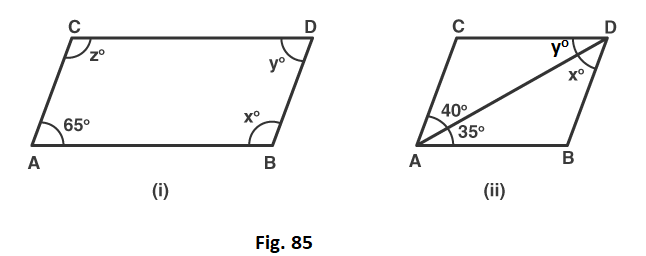Solution:

(i)  Since, AC ∥ BD and CD ∥ AB, ABCD is a parallelogram

∠CAB + ∠ACD = 180o

∠ACD = 180o – 65o

= 115o

Opposite angles of parallelogram,

∠CAB = ∠CDB = 65o

∠ACD = ∠DBA = 115o

(ii)  Here,

AC ∥ BD and CD ∥ AB

Alternate interior angles,

∠DAB = y = 35o

29. In Fig. 86, state which lines are parallel and why?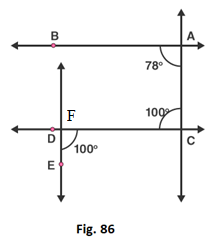Solution:

Let, F be the point of intersection of the line CD and the line passing through point E.

Here, ∠ACD and ∠CDE are alternate and equal angles.

So, ∠ACD = ∠CDE = 100o

Therefore, AC ∥ EF

30. In Fig. 87, the corresponding arms of ∠ABC and ∠DEF are parallel. If ∠ABC = 75o, find ∠DEF.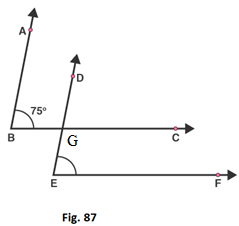Solution:

Let, G be the point of intersection of the lines BC and DE

Since, AB ∥ DE and BC ∥ EF

The corresponding angles are,

∠ABC = ∠DGC = ∠DEF = 75o